# Uniform star polyhedron

﻿
Uniform star polyhedron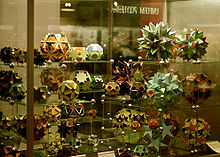A display of uniform polyhedra at the Science Museum in London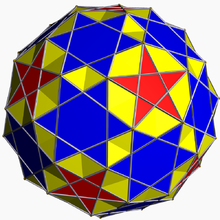The small snub icosicosidodecahedron is a uniform star polyhedron, with vertex figure 35.5/2

In geometry, a uniform star polyhedron is a self-intersecting uniform polyhedron. They are also sometimes called nonconvex polyhedra to imply self-intersecting. Each polyhedron can contain either star polygon faces, star polygon vertex figures or both.

The complete set of 57 nonprismatic uniform star polyhedra includes the 4 regular ones, called the Kepler–Poinsot polyhedra, 5 quasiregular ones, and 48 semiregular ones.

There are also two infinite sets of uniform star prisms and uniform star antiprisms.

Just as (nondegenerate) star polygons (which have Polygon density greater than 1) correspond to circular polygons with overlapping tiles, star polyhedra that do not pass through the center have polytope density greater than 1, and correspond to spherical polyhedra with overlapping tiles; there are 48 nonprismatic such uniform star polyhedra. The remaining 9 nonprismatic uniform star polyhedra, those that pass through the center, are the hemipolyhedra, and do not correspond to spherical polyhedra, as the center cannot be projected uniquely onto the sphere.

The nonconvex forms are constructed from Schwarz triangles.

All the uniform polyhedra are listed below by their symmetry groups and subgrouped by their vertex arrangements.

Regular polyhedra are labeled by their Schläfli symbol. Other nonregular uniform polyhedra are listed with their vertex configuration or their Uniform polyhedron index U(1-80).

Note: For nonconvex forms below an additional descriptor Nonuniform is used when the convex hull vertex arrangement has same topology as one of these, but has nonregular faces. For example an nonuniform cantellated form may have rectangles created in place of the edges rather than squares.

## Tetrahedral symmetry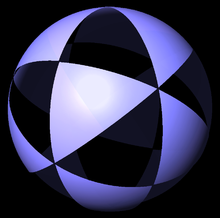(3 3 2) triangles on sphere

There are two nonconvex forms, the tetrahemihexahedron and octahemioctahedron which have tetrahedral symmetry (with fundamental domain Mobius triangle (3 3 2)).

There are two Schwarz triangles that generate unique nonconvex uniform polyhedra: one right triangle (3/2 3 2), and one general triangle (3/2 3 3).

## Octahedral symmetry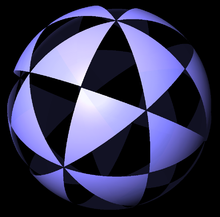(4 3 2) triangles on sphere

There are 8 convex forms, and 10 nonconvex forms with octahedral symmetry (with fundamental domain Mobius triangle (4 3 2)).

There are four Schwarz_triangles that generate nonconvex forms, two right triangles (3/2 4 2), and (4/3 3 2), and two general triangles: (4/3 4 3), (3/2 4 4).

## Icosahedral symmetry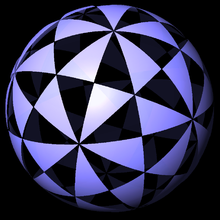(5 3 2) triangles on sphere

There are 8 convex forms and 46 nonconvex forms with icosahedral symmetry (with fundamental domain Mobius triangle (5 3 2)). (or 47 nonconvex forms if Skilling's figure is included). Some of the nonconvex snub forms have reflective vertex symmetry.

### Skilling's figure

One further nonconvex polyhedron is the Great disnub dirhombidodecahedron, also known as Skilling's figure, which is vertex-uniform, but has pairs of edges which coincide in space such that four faces meet at some edges. It is sometimes but not always counted as a uniform polyhedron. It has Ih symmetry.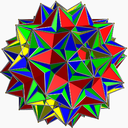## Degenerate cases

Coxeter identified a number of degenerate star polyhedra by the Wythoff construction method, which contain overlapping edges or vertices. These degenerate forms include:

Wikimedia Foundation. 2010.

### Look at other dictionaries:

• Star polyhedron — In geometry, a star polyhedron is a polyhedron which has some repetitive quality of nonconvexity giving it a star like visual quality.There are two general kinds of star polyhedron: *Polyhedra which self intersect in a repetitive way. *Concave… …   Wikipedia

• Polyhedron — Polyhedra redirects here. For the relational database system, see Polyhedra DBMS. For the game magazine, see Polyhedron (magazine). For the scientific journal, see Polyhedron (journal). Some Polyhedra Dodecahedron (Regular polyhedron) …   Wikipedia

• Star polygon — Set of regular star polygons {5/2} {7/2} …   Wikipedia

• Uniform polychoron — In geometry, a uniform polychoron (plural: uniform polychora) is a polychoron or 4 polytope which is vertex transitive and whose cells are uniform polyhedra.This article contains the complete list of 64 non prismatic convex uniform polychora, and …   Wikipedia

• Uniform polyhedron — A uniform polyhedron is a polyhedron which has regular polygons as faces and is transitive on its vertices (i.e. there is an isometry mapping any vertex onto any other). It follows that all vertices are congruent, and the polyhedron has a high… …   Wikipedia

• Uniform polytope — A uniform polytope is a vertex transitive polytope made from uniform polytope facets. A uniform polytope must also have only regular polygon faces.Uniformity is a generalization of the older category semiregular, but also includes the regular… …   Wikipedia

• Nonconvex uniform polyhedron — In geometry, a nonconvex uniform polyhedron, or uniform star polyhedron, is a self intersecting uniform polyhedron. Each can contain either star polygon faces, star polygon vertex figures or both.The complete set of 57 nonprismatic uniform star… …   Wikipedia

• Omnitruncated polyhedron — In geometry, an omnitruncated polyhedron is a truncated quasiregular polyhedron. When they are alternated, they produce the snub polyhedra. All omnitruncated polyhedra are zonohedra. They have Wythoff symbol p q r | and vertex figures as 2p.2q.2r …   Wikipedia

• List of Wenninger polyhedron models — This table contains an indexed list of the Uniform and stellated polyhedra from the book Polyhedron Models , by Magnus Wenninger.The book was written as a guide book to building polyhedra as physical models. It includes templates of face elements …   Wikipedia

• List of uniform polyhedra — Uniform polyhedra and tilings form a well studied group. They are listed here for quick comparison of their properties and varied naming schemes and symbols.This list includes: * all 75 nonprismatic uniform polyhedra; * a few representatives of… …   Wikipedia